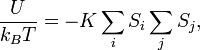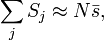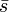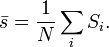# Mean field models

(diff) ← Older revision | Latest revision (diff) | Newer revision → (diff)

A mean field model, or a mean field solution of a model, is an approximation to the actual solution of a model in statistical physics. The model is made exactly solvable by treating the effect of all other particles on a given one as a mean field (hence its name). It appear in different forms and different contexts, but all mean field models have this feature in common.

## Mean field solution of the Ising model

A well-known mean field solution of the Ising model goes as follows. From the original hamiltonian,$\frac{U}{k_B T} = - K \sum_i S_i \sum_j S_j ,$

suppose we may approximate$\sum_j S_j \approx N \bar{s},$

where$N$ is the number of neighbors of site$i$ (e.g. 4 in a 2-D squate lattice), and$\bar{s}$ is the (unknown) magnetization:$\bar{s}=\frac{1}{N} \sum_i S_i .$